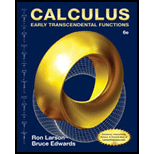Chapter 15.2, Problem 21ECalculus: Early Transcendental Fun...

6th Edition
Ron Larson + 1 other
ISBN: 9781285774770

Solutions

Chapter
SectionCalculus: Early Transcendental Fun...

6th Edition
Ron Larson + 1 other
ISBN: 9781285774770
Textbook Problem

Mass In Exercises 23 and 24, find the total mass of a spring with density ρ in the shape of the circular helix r ( t ) = 2 cos t i + 2 sin t j + t k , 0 ≤ t ≤ 4 π ⋅ ρ ( x , y , z ) = 1 2 ( x 2 + y 2 + z 2 )

To determine

To calculate: The total mass of a spring with density ρ if the spring has a density ρ(x,y,z)=12(x2+y2+z2) in the shape of the circular helix given by r(t)=2costi+2sintj+tk; 0t4π

Explanation

Given:

The spring has a density ρ(x,y,z)=12(x2+y2+z2) in the shape of the circular helix given by r(t)=2costi+2sintj+tk; 0t4π

Formula used:

For parameterization given by r(t)=xi+yj+zk, the length of curve ds is,

ds=r(t)dt

Calculation:

For the curve r(t)=2costi+2sintj+tk, x(t)=2cost, y(t)=2sint, and z(t)=t which implies that x(t)=2sint, y(t)=2cost, and z(t)=1. We can use x(t), y(t) and z(t) to find ds,

ds=r(t)dt=x(t)2+y(t)2+z(t)2dt=(2sint)2+(2cost)2+12dt=

Still sussing out bartleby?

Check out a sample textbook solution.

See a sample solution

The Solution to Your Study Problems

Bartleby provides explanations to thousands of textbook problems written by our experts, many with advanced degrees!

Get Started

Simplify the expressions in Exercises 97106. (xy)1/3(yx)1/3

Finite Mathematics and Applied Calculus (MindTap Course List)

Find the limit. 44. limni=1n1n[(in)3+1]

Single Variable Calculus: Early Transcendentals, Volume I

In problems 5-8, write the following sets in a second way. 6.

Mathematical Applications for the Management, Life, and Social Sciences

Evaluate the integral. 17. xcoshxdx

Single Variable Calculus: Early Transcendentals